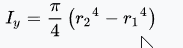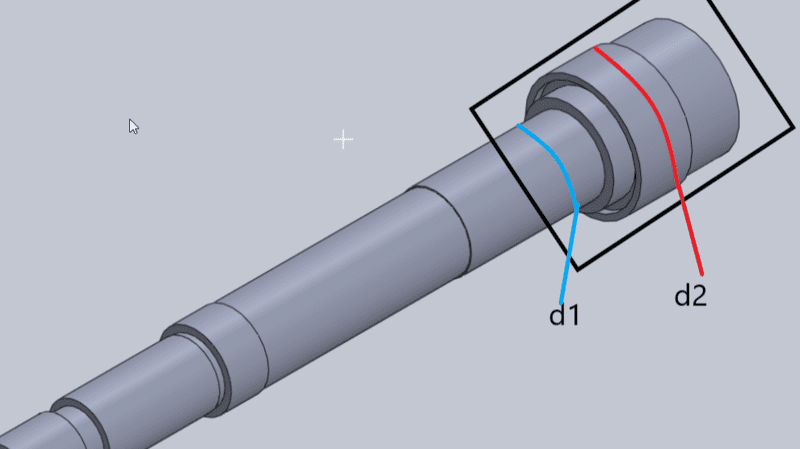# How to add area moments of inertia for varying cross sections

friscoboya
Hello

I am doing a shaft design layout for a spindle to minimize deflection and I have to calculate the area moment of inertia for a shaft overhang.The shaft overhang is the distance from a bearing to the end of the shaft. Here is a picture detailing the location of the overhang. The black square would be the shaft overhang. Would I calculate the area moment of inertia for d1 and d2, add them together and average them? Or what is the correct method to add area moment of inertias? The equation for hollow cylinder area moment of inertia is.Thanks

#### Attachments

Homework Helper
Gold Member
It depends what you are going to do with the area moment of inertia. How are you going to use it?

friscoboya
It depends what you are going to do with the area moment of inertia. How are you going to use it?
So the shaft design would be for a CNC machine primarily used for milling. The area moment of inertia would be put into an equation I found from papers. Area moment of inertia is used for deflection calculation which is what I am focusing on. Yeah whats confusing me is the different diameters, it would be simple if the diameters were constant throughout the shaft overhang (black box).

N P
If you have access to Mechanical Engineering Design by Shigley-Mischke-Budynas, it shows you how the deflection should be calculated. You have to perform some integration. I find it quite complicated.
.
However, if you want to calculate a "limiting," or maximum possible deflection, you can assume the entire shaft has diameter d1 - the smaller diameter. This is often a more practical approach, but of course it depends on your application.

Another website is-
https://www.machinedesign.com/archive/calculate-deflection-stepped-shafts-easy-way

Still not easy in my opinion.

•berkeman